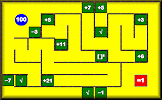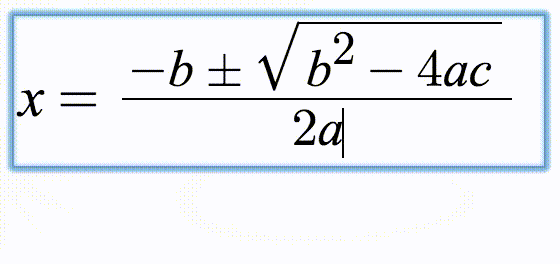# Differentiation

## Practise the technique of differentiating polynomials and other functions with this self marking exercise.

##### Menu  Level 1L 2L 3L 4L 5L 6L 7L 8L 9L 10L 11  Exam    Help

This is level 1: differentiate basic polynomials. You can earn a trophy if you get at least 9 questions correct and you do this activity online. Use the ^ key to type in a power or index and use the forward slash / to type a fraction. Press the right arrow key to end the power or fraction. Click the Help tab above for more.

 $$y=x^{4}$$ $$\frac{dy}{dx}=$$$$y=x^{3}$$ $$\frac{dy}{dx}=$$$$y=x^{2}$$ $$\frac{dy}{dx}=$$$$y=x$$ $$\frac{dy}{dx}=$$$$y=x^{3}+x^{2}$$ $$\frac{dy}{dx}=$$$$y=x^{4}-x^{3}$$ $$\frac{dy}{dx}=$$$$y=x^{2}+9$$ $$\frac{dy}{dx}=$$$$y=8x^{2}$$ $$\frac{dy}{dx}=$$$$y=3x$$ $$\frac{dy}{dx}=$$$$y=3$$ $$\frac{dy}{dx}=$$$$y=4x^{3}+2x^{2}-3$$ $$\frac{dy}{dx}=$$$$y=9x^{3}-6x^{2}+8$$ $$\frac{dy}{dx}=$$Check

This is Differentiation level 1. You can also try:
Level 2 Level 3 Level 4 Level 5 Level 6 Level 7 Level 8 Level 9 Level 10 Level 11

## Instructions

Try your best to answer the questions above. Type your answers into the boxes provided leaving no spaces. As you work through the exercise regularly click the "check" button. If you have any wrong answers, do your best to do corrections but if there is anything you don't understand, please ask your teacher for help.

When you have got all of the questions correct you may want to print out this page and paste it into your exercise book. If you keep your work in an ePortfolio you could take a screen shot of your answers and paste that into your Maths file.

## Transum.org

This web site contains over a thousand free mathematical activities for teachers and pupils. Click here to go to the main page which links to all of the resources available.## More Activities:

Mathematicians are not the people who find Maths easy; they are the people who enjoy how mystifying, puzzling and hard it is. Are you a mathematician?

Comment recorded on the 5 April 'Starter of the Day' page by Mr Stoner, St George's College of Technology:

"This resource has made a great deal of difference to the standard of starters for all of our lessons. Thank you for being so creative and imaginative."

Comment recorded on the 6 May 'Starter of the Day' page by Natalie, London:

"I am thankful for providing such wonderful starters. They are of immence help and the students enjoy them very much. These starters have saved my time and have made my lessons enjoyable."

Each month a newsletter is published containing details of the new additions to the Transum website and a new puzzle of the month.

The newsletter is then duplicated as a podcast which is available on the major delivery networks. You can listen to the podcast while you are commuting, exercising or relaxing.

Transum breaking news is available on Twitter @Transum and if that's not enough there is also a Transum Facebook page.

#### BidmazeFind your way through the maze encountering mathematical operations in the correct order to achieve the given total. This is an addictive challenge that begins easy but develops into quite a difficult puzzle.

There are answers to this exercise but they are available in this space to teachers, tutors and parents who have logged in to their Transum subscription on this computer.

A Transum subscription unlocks the answers to the online exercises, quizzes and puzzles. It also provides the teacher with access to quality external links on each of the Transum Topic pages and the facility to add to the collection themselves.

Subscribers can manage class lists, lesson plans and assessment data in the Class Admin application and have access to reports of the Transum Trophies earned by class members.

Subscribe

## Go Maths

Learning and understanding Mathematics, at every level, requires learner engagement. Mathematics is not a spectator sport. Sometimes traditional teaching fails to actively involve students. One way to address the problem is through the use of interactive activities and this web site provides many of those. The Go Maths page is an alphabetical list of free activities designed for students in Secondary/High school.

## Maths Map

Are you looking for something specific? An exercise to supplement the topic you are studying at school at the moment perhaps. Navigate using our Maths Map to find exercises, puzzles and Maths lesson starters grouped by topic.

## Teachers

If you found this activity useful don't forget to record it in your scheme of work or learning management system. The short URL, ready to be copied and pasted, is as follows:

Alternatively, if you use Google Classroom, all you have to do is click on the green icon below in order to add this activity to one of your classes.

It may be worth remembering that if Transum.org should go offline for whatever reason, there are mirror sites at Transum.com and Transum.info that contain most of the resources that are available here on Transum.org.

When planning to use technology in your lesson always have a plan B!

Richard, Pennsylvania

Sunday, October 9, 2022

"Level 7:
y= sqrt(5x^2 -8x)
The answer is (5x-4)/sqrt(5x^2-8x) but for some reason it is marked as wrong. I want to confirm that this isn't an error or anything. Thanks!

[Transum: Thanks for your feedback Richard. You are right. The software stores a number of different versions of the correct answer and the format you chose was missing. I have now corrected this error. Sorry for the inconvenience and thanks again for taking the time to let me know.]"

Do you have any comments? It is always useful to receive feedback and helps make this free resource even more useful for those learning Mathematics anywhere in the world. Click here to enter your comments.For All:

© Transum Mathematics :: This activity can be found online at:
www.Transum.org/go/?Num=55

## Description of Levels

CloseBefore beginning these exercises make sure you understand Indices really well.

You should also have been shown how to differentiate from first principles.

Level 1 - Differentiate basic polynomials

Level 2 - Differentiate polynomials including negative and fractional indices

Level 3 - Calculations involving the gradient at the given point

Level 4 - Finding tangents and normals

Level 5 - Differentiate trigonometric functions

Level 6 - Differentiate exponential and natural logarithm functions

Level 7 - Differentiate using the chain rule

Level 8 - Differentiate using the product rule

Level 9 - Differentiate using the quotient rule

Level 10 - Interpreting derivatives and second derivatives, maxima, minima and points of inflection.

Level 11 - Differentiate simple functions parametrically

Exam Style questions are in the style of IB or A-level exam paper questions and worked solutions are available for Transum subscribers.

Integration - Exercises on indefinite and definite integration of basic algebraic and trigonometric functions.

Answers to this exercise are available lower down this page when you are logged in to your Transum account. If you don’t yet have a Transum subscription one can be very quickly set up if you are a teacher, tutor or parent.

## Mathematical Notation

Use the ^ key to type in a power or index then the right arrow or tab key to end the power.

For example: Type 3x^2 to get 3x2.

Use the forward slash / to type a fraction then the right arrow or tab key to end the fraction.

For example: Type 1/2 to get ½.

Fractions should be given in their lowest terms.

A square root sign (if required) should be typed in as \sqrt space then press the right arrow key after typing in the last term in the square root.

### Typing Mathematical Notation

These exercises use MathQuill, a web formula editor designed to make typing Maths easy and beautiful. Watch the animation below to see how common mathematical notation can be created using your keyboard.## Terminology and symbols

Please note that if $$y = f(x) = x^2$$ then the first differential can be shown in any of the following ways:

$$\frac{dy}{dx} = 2x$$ $$y' = 2x$$ $$f'(x) = 2x$$

## Differentiating Trigonometric Functions

$$\frac{d}{dx} (\sin x) = \cos x$$ $$\frac{d}{dx} (\cos x) = -\sin x$$ $$\frac{d}{dx} (\tan x) = \frac{1}{\cos^2 x}$$

## Differentiating Other Functions

$$\frac{d}{dx} (e^x) = e^x$$ $$\frac{d}{dx} ( \ln x) = \frac{1}{x}$$

In the following rules, $$u$$ and $$v$$ are functions of $$x$$.

## The Product Rule

$$\text{If} \quad y = uv \quad \text{then}$$ $$\frac{dy}{dx} = v\frac{du}{dx} + u\frac{dv}{dx}$$

## The Quotient Rule

$$\text{If} \quad y = \frac{u}{v} \quad \text{then}$$ $$\frac{dy}{dx} = \frac{v\frac{du}{dx} - u\frac{dv}{dx}}{v^2}$$

## The Chain Rule

$$\frac{dy}{dx} = \frac{dy}{du} \times \frac{du}{dx}$$